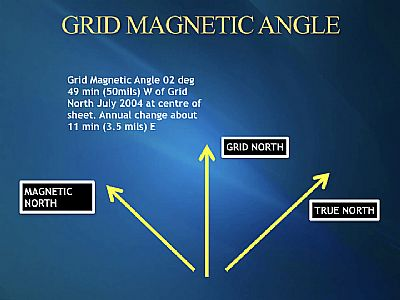## GRID MAGNETIC ANGLE

This information is found at the top centre of the map and is required so the the Grid Magnetic Angle can be calculated for the current year.

It is used when converting Grid and Magnetic Bearings.How to calculate the Grid Magnetic Angle (GMA).

• GMA 02deg 58 min West of grid North, July 2017.
• Annual change 14 min East.
• Take the curent year in this case 2018 subtract 2017 =1 year.
• Remember the annual change was 14 min east.
• Multiply this with the total year difference 14 min x 1 year =14 min.
• Subtract the 14 min from the GMA 02 deg 58 min = 2deg 44 min
• Round this up to the whole figure, and the the GMA you would use for 2018 would be 3 deg.

Example 2.

• GMA of 5deg 30 min West of Grid North 2003, annual change 09 min east.
• Take the curent year in this case 2018 subtract 2003 = 15 years.
• Remember annual change 9 min east.
• Multiply this with total years 9min x 15 years =135 min.
• Divide the amount 135 min by 60 this will give you 2 deg 15min.
• Subtract the 2 deg 15 min from the GMA 5deg 30 min = 3 deg 15min.
• Round this up to the nearest whole figure in this case 3 deg, and the GMA you would use for 2018 would be 3 deg.

Note: If it hade been 3 deg 35 min you would have gone to the nearest whole fig which would have been 4 deg.

You will have noticed in both examples there was a big difference in the years ie 1 year and 15 years but once calculated for the current year ie 2018 we came up with the same GMA of 3 deg.